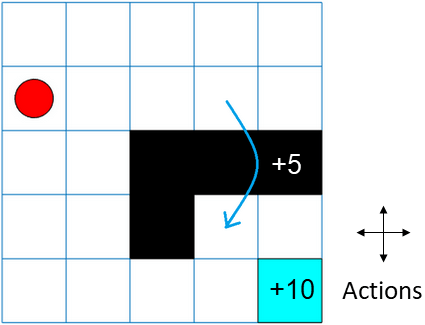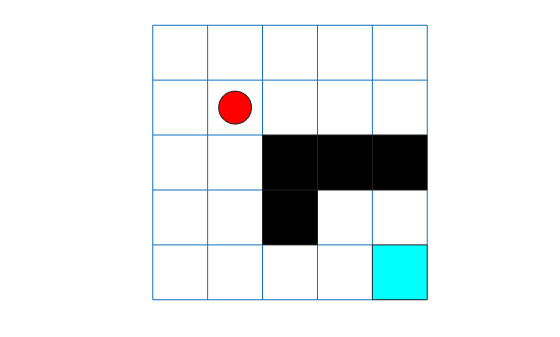rlMDPEnv

Create Markov decision process environment for reinforcement learning

Description

A Markov decision process (MDP) is a discrete time stochastic control process. It provides a mathematical framework for modeling decision making in situations where outcomes are partly random and partly under the control of the decision maker. MDPs are useful for studying optimization problems solved using reinforcement learning. Use rlMDPEnv to create a Markov decision process environment for reinforcement learning in MATLAB®.

Creation

Description

example

env = rlMDPEnv(MDP) creates a reinforcement learning environment env with the specified MDP model.

Input Arguments

expand all

Markov decision process model, specified as one of the following:

Properties

expand all

Markov decision process model, specified as a GridWorld object or GenericMDP object.

Reset function, specified as a function handle.

Object Functions

 getActionInfo Obtain action data specifications from reinforcement learning environment or agent getObservationInfo Obtain observation data specifications from reinforcement learning environment or agent sim Simulate trained reinforcement learning agents within specified environment train Train reinforcement learning agents within a specified environment validateEnvironment Validate custom reinforcement learning environment

Examples

collapse all

For this example, consider a 5-by-5 grid world with the following rules:

1. A 5-by-5 grid world bounded by borders, with 4 possible actions (North = 1, South = 2, East = 3, West = 4).

2. The agent begins from cell [2,1] (second row, first column).

3. The agent receives reward +10 if it reaches the terminal state at cell [5,5] (blue).

4. The environment contains a special jump from cell [2,4] to cell [4,4] with +5 reward.

5. The agent is blocked by obstacles in cells [3,3], [3,4], [3,5] and [4,3] (black cells).

6. All other actions result in -1 reward.First, create a GridWorld object using the createGridWorld function.

GW = createGridWorld(5,5)
GW =
GridWorld with properties:

GridSize: [5 5]
CurrentState: "[1,1]"
States: [25x1 string]
Actions: [4x1 string]
T: [25x25x4 double]
R: [25x25x4 double]
ObstacleStates: [0x1 string]
TerminalStates: [0x1 string]

Now, set the initial, terminal and obstacle states.

GW.CurrentState = '[2,1]';
GW.TerminalStates = '[5,5]';
GW.ObstacleStates = ["[3,3]";"[3,4]";"[3,5]";"[4,3]"];

Update the state transition matrix for the obstacle states and set the jump rule over the obstacle states.

GW.T(state2idx(GW,"[2,4]"),:,:) = 0;
GW.T(state2idx(GW,"[2,4]"),state2idx(GW,"[4,4]"),:) = 1;

Next, define the rewards in the reward transition matrix.

nS = numel(GW.States);
nA = numel(GW.Actions);
GW.R = -1*ones(nS,nS,nA);
GW.R(state2idx(GW,"[2,4]"),state2idx(GW,"[4,4]"),:) = 5;
GW.R(:,state2idx(GW,GW.TerminalStates),:) = 10;

Now, use rlMDPEnv to create a grid world environment using the GridWorld object GW.

env = rlMDPEnv(GW)
env =
rlMDPEnv with properties:

Model: [1x1 rl.env.GridWorld]
ResetFcn: []

You can visualize the grid world environment using the plot function.

plot(env)Introduced in R2019a